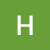# Solving Popular Algorithms: Matrix Diagonal Sums

`mat = [[1,2,3],[4,5,6],[7,8,9]]`
`diagonalSum = (mat) => {   let primSum = 0      for (let i = 0; i < mat.length; i++) {      primSum += mat[i][i]   } }`
`diagonalSum = (mat) => {   let primSum = 0   let secSum = 0   for (let i = 0; i < mat.length; i++) {      primSum += mat[i][i]      secSum += mat[i][mat.length-1-i]   } }`
`diagonalSum = (mat) => {   let primSum = 0   let secSum = 0   for (let i = 0; i < mat.length; i++) {      primSum += mat[i][i]      secSum += mat[i][mat.length-1-i]   }      if (mat.length%2 !== 0) {      let mid = Math.floor(mat.length/2)      secSum -= mat[mid][mid]   }      return primSum + secSum}`

--

--

--

## More from HopeGiometti

Love podcasts or audiobooks? Learn on the go with our new app.

## Epns AMA with AlphaFinanceLab## Creating Postman Tests for API Requests## Transition options to SAP S/4HANA## R-Planet guide to Uplift your land!## Build a Twitter Bot with Node.js and the Pokémon API## Hedgehog Team Development for Sitecore (TDS) v5.8 Review## 3-D Printed Robotic Arm## My Experience With Debugging## Where Visuals And Algorithms Collide## Guide To Dynamic Programming 👨‍💻## Git Rebase vs Git Merge